NCERT Solutions for Class 7 Maths Chapter 13 Exponents and Powers Ex 13.2 are part of NCERT Solutions for Class 7 Maths. Here we have given NCERT Solutions for Class 7 Maths Chapter 13 Exponents and Powers Ex 13.2.

 Board CBSE Textbook NCERT Class Class 7 Subject Maths Chapter Chapter 13 Chapter Name Exponents and Powers Exercise Ex 13.2 Number of Questions Solved 5 Category NCERT Solutions

## NCERT Solutions for Class 7 Maths Chapter 13 Exponents and Powers Ex 13.2

Question 1.
Using laws of exponents, simplify and write the answer in exponential form :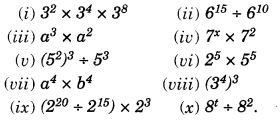Solution: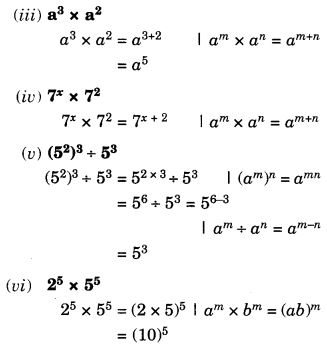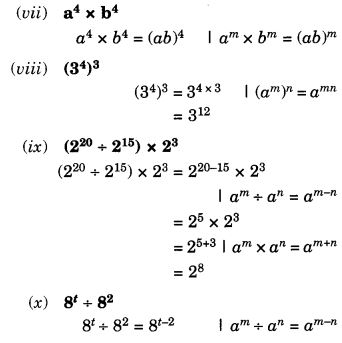Question 2.
Simplify and express each of the following in exponential form:Solution: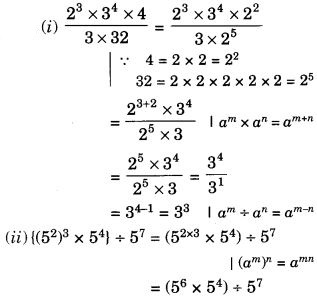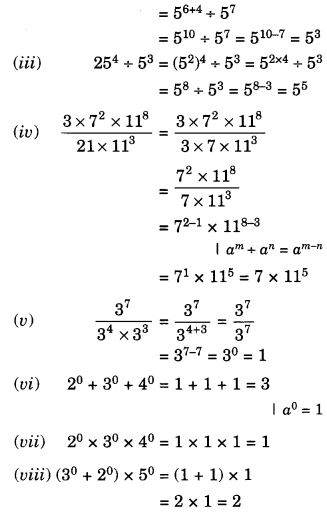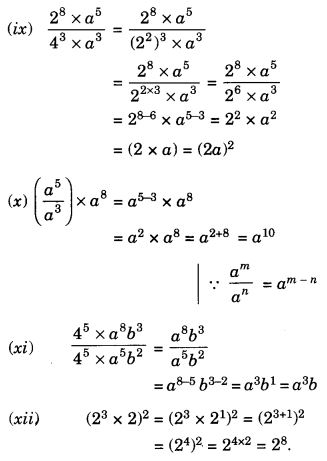Question 3.Solution: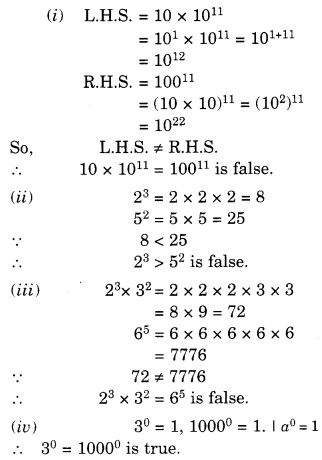Question 4.
Express each of the following as a product of prime factors only in exponential form:
(i) 108 × 192
(ii) 270
(iii) 729 × 64
(iv) 768.
Solution: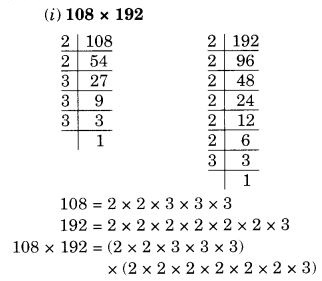Question 5.
Simplify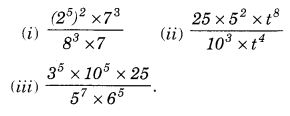Solution: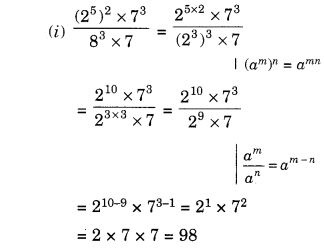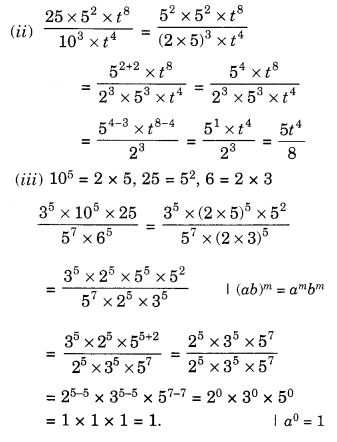We hope the NCERT Solutions for Class 7 Maths Chapter 13 Exponents and Powers Ex 13.2 help you. If you have any query regarding NCERT Solutions for Class 7 Maths Chapter 13 Exponents and Powers Ex 13.2, drop a comment below and we will get back to you at the earliest.